# Chemical Kinetics - Reaction Rates | Chemical Kinetics Help

## What Is Chemical Kinetics?

Chemical kinetics, also known as reaction kinetics, is the study of rates of chemical processes with respect to reaction rates, effect of various variables, re-arrangement of atoms, formation of intermediates etc.

### Rate of Reaction:

Rates of reactions are the rates of change in concentrations or amounts of either reactants or products. Thermodynamics and kinetics are two factors that affect rate of reactions.With the progress of reaction the concentration of reactants decreases while that (those) of product(s) increases. If the reaction occurs quickly, the concentration (mole L–1) of reactant will decrease rapidly while that of product will increase rapidly. Thus, the rate of reaction is defined as the rate at which the concentration of reactant decreases or alternatively, the rate at which concentration of product increases. That is the change in concentration of any of the substance (reactant/product) per unit time during the reaction is called rate of reaction. If DC be the change in concentration of any reactant or any product during the time interval Dt then the rate may be given as Rate = ±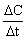(– ve sign applies in the case of reactant whose concentration goes on decreasing with time and + ve sign applies in the case of product whose concentration goes on increasing with time).

However, the rate of reaction is not uniform. With the passage of time the concentration(s) of reactant(s) go(es) on decreasing and hence according to Law of Mass Action, the rate of reaction goes on decreasing. Infact, the rate of reaction decreases moment to moment. This is shown in the graph of rate vs time curve of a reaction.

Rate varies from moment to moment so rate of reaction has to be specified at a given instant of time and it is called instantaneous rate or rate at any time t. This is as defined below.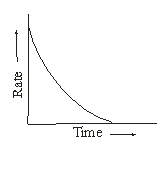rinst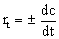Where dc is the infinitesimal change in concentration during infinitesimal time interval dt after time t i..e. between t and t + dt. The time interval dt being infinitesimal small, the rate of reaction may be assumed constant during the interval.

The rate of reaction expressed as ± DC/Dt is actually the average rate with time interval considered.

For the reaction; 2N2O5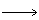4NO2 + O2

The rate of reaction at any time t may be expressed by one of the following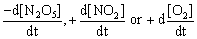Where square bracket terms denote molar concentration of the species enclosed.

The above three rates are not equal to one another as is evident from the stoichiometry of the reaction. For every mole of N2O5 decomposed, 2 moles NO2 and 1/2 mole of O2 will be formed. Obviously, the rate of formation of NO2 will be four times that of O2 and it is twice the rate of consumption of N2O5. Thus, their three rates are interrelated as,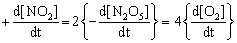Dividing through out by 4,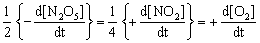Thus, rate of reaction expressed in terms of the various species involved in a reaction will be equal to one another if each of them is divided by the stoichiometric constituent of the species concerned. Rate divided by stoichiometric coefficient may be called as rate per mole.

The kinetic experiment has shown that the rate of reaction mentioned above increases same number of times as the number of times the concentration of N2O5 is increased. That is, rate is doubled by doubling the concentration of N2O5. This may be mathematically expressed as

Rate µ [N2O5]

or Rate = k [N2O5] …(1)

Where k is the proportionality constant called rate constant, velocity coefficient or specific reaction rate of the reaction, and is a constant for a given reaction at a given temperature. Equation 1 showing the concentration – dependence of rate is called rate law of the reaction. Expressing rate in terms of change of concentration of various species, equation may be put as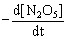= k¢ [N2O5]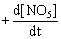= k² [NO2]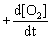= k²¢ [O2]

when k¢, k² and k²¢ are the rate constants of the reaction. These three rate constants are inter-related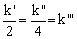Thus, for a reaction represented by the general equation

aA + bBcC + dD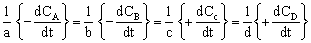We have,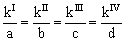where

kI, kII, kIII and kIV

are the rate constants of the reaction when its rate is expressed in terms A, B, C and D, respectively. Units of rate are given below:

Unit of rate =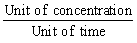= Concentration time–1 i.e. mole L–1 S–1### Email Based Assignment Help in Chemical Kinetics

Assignmenthelp.net - A leading online Chemistry Assignment Help provider in Chemistry and Chemical Kinetics. Find answers to all of your doubts regarding chemical kinetics in chemistry. We provide chemistry homework, Assignment Help to the school, college or university level students. Our expert online tutors are available to help you with chemical kinetics, rate of reactions, online chemistry, thermodynamics and kinetics problems. Our Chemistry Assignment Help Service is focused on: on time delivery, superior quality, fine creativity, originality and plagiarism free.